## LetsPlayMaths.Com

WELCOME TO THE WORLD OF MATHEMATICS

# Class 2 Multiplication Worksheet - 3

1. One apple costs Rs. 9. How much 8 apples will cost?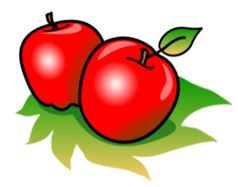2. One football team has 11 players. How many players are there in 6 teams?3. John bought 5 balls for Rs. 7 each. How much did he pay?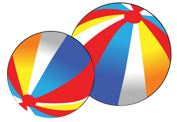4. A packet has 10 pens. How many pens would be there in 8 packets?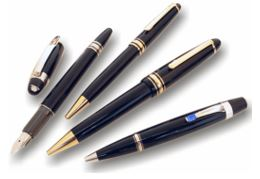5. A sportsman plays 8 hours a day. How many hours does he play in a week?6. One packet can contain 9 oranges. How many oranges will be there in 7 such packets?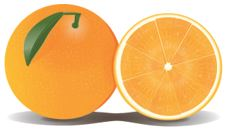7. One eraser costs Rs. 5. How much 6 erasers will cost?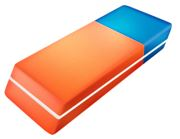8. In a school bus 12 students can travel. How many students can travel in 6 similar buses?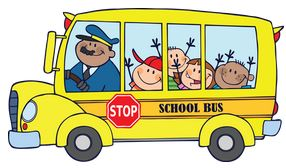9. A box has 12 sweets. How many sweets are there in 9 boxes?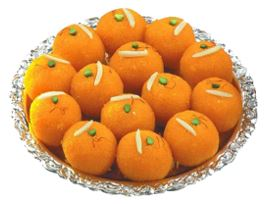10. Stacy bought 5 dolls for Rs. 15 each. How much did she pay?11. 14 x _____ = 140.

12. 12 x 9 = ________.

13. 15 x 7 = ________.

14. 15 x 10 = 5 x 30. Mark True / False.

15. 11 x 9 = 30 x 3. Mark True / False.

16. 70 = 5 x _____.

17. 13 x _____ = 52.

18. 8 x 13 = ________.

19. 145 = 15 x 9. Mark True / False.

20. 14 x 9 > 130. Mark True / False.

Multiplication Worksheet - 1

Multiplication Worksheet - 2

Multiplication Worksheet - 3